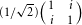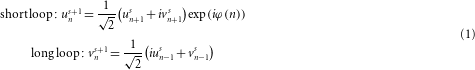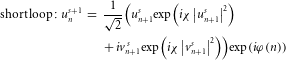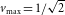## Main

Newton’s third law states that action–reaction forces involved in an elemental two-body interaction must be equal and opposite: F1 = −F2. Combined with the second law of motion, this leads to m1a1 = −m2a2, where m1,2 represent the masses of these two particles and a1,2 their respective accelerations. Given that mass is by nature a positive quantity, two classical bodies are expected to accelerate either towards or away from each other, but never in the same direction (Fig. 1a). However, this situation completely changes if hypothetically one of the masses is negative. In fact, in this regime, an intriguing scenario arises whenever m1 = −m2; under these conditions, two interacting bodies will indefinitely accelerate in the same direction while keeping a constant distance among themselves (Fig. 1a). Interestingly, this possibility was first speculated within the context of diametric drive that could itself provide a possible mechanism for space propulsion1. Of course, given that in classical mechanics the mass of a particle is always positive, no such acceleration behaviour that breaks the action–reaction symmetry has ever been reported.Figure 1: Diametric drive interaction between two particles, fibre-optic set-up and mesh lattice dispersion (effective mass) diagram.

Waves on the other hand are free of such limitations. In the case of a free quantum particle governed by the Schrödinger equation, the resulting parabolic dispersion relation between energy and momentum has a positive curvature defined by the particle’s mass. Meanwhile, quasiparticles such as electrons and holes in solid-state crystals, mass–spring systems5 or collective excitations such as Bose–Einstein condensates in lattices6,7 may exhibit a dispersion relation with regions of inverted curvature where the effective mass is negative. Similarly, in photonic guiding structures, the effective photon mass can be positive or negative depending on the sign of the associated group velocity dispersion3,4. In addition, photonic lattices such as waveguide arrays15,16 or mesh lattices8,9,10,11,12 provide a versatile environment to experimentally investigate this class of phenomena, as broad light excitations of these structures are effectively governed by their respective mass that can be either positive or negative (Fig. 1c). The second component needed for realizing a diametric drive is an effective interaction between the two entities involved. Although in general such forces can emerge from a number of physical processes, in optics, this can be achieved only through nonlinear effects. For example in waveguide structures, optical wave packets can interact through third-order Kerr nonlinearity—in direct analogy to point-like scattering in Bose–Einstein condensates6,7,17. In this setting, an effective force is established between two partially overlapping light beams2,18,19. Therefore, nonlinear optical periodic configurations can serve as an ideal platform to observe diametric drive acceleration and to study action–reaction symmetry breaking effects.

Here we experimentally demonstrate such optical self-accelerating bound states in a temporal mesh lattice. A composite beam or a wave packet is formed by a sequence of circulating optical pulses11,12 propagating in two fibre loops connected by a 50/50 coupler10 (Fig. 1b and Supplementary Methods). A length difference ΔL between the two loops essentially creates an effective grid of positions or time slots n that are coupled to their nearest neighbours at every propagation step or loop round-trip s. A light pulse propagating in the short loop takes a shortcut and thus advances by a fixed time amount, moving its time slot to the left from n+1 to n. In contrast, a pulse circulating in the long loop is delayed from n−1 to n. After each round trip s, pulse sequences in both loops are linearly interfered by the matrixof the central 50/50 coupler. In the linear optical regime of low pulse powers, this results in the following system of coupled evolution equations10:Here, uns and vns are the pulse amplitudes at the time slot or position n and propagation step s circulating in the short and long loop, respectively. The term φ(n) denotes the external phase shift being imprinted onto the field during each round trip by a phase modulator in the short loop. Similar to cases where a refractive index distribution is present, this phase accumulates during evolution and affects the interference between neighbouring pulses. Hence, φ(n) can be regarded as a position-dependent effective potential.

The required interaction between optical pulses is provided by the Kerr nonlinearity of the optical fibres themselves. The pulse powers needed to induce these nonlinear effects are sustained by employing erbium-doped fibre amplifiers that compensate for round-trip losses.

In our set-up, the resulting nonlinear pulse dynamics are described by14:where the coefficient χ represents the accumulated nonlinearity in each loop (Supplementary Methods).

As previously indicated, the arrangement used here is in every respect equivalent to a periodic mesh lattice and as such it exhibits a band structure8,10. The corresponding dispersion relation is obtained by using discrete Floquet–Bloch modes (uns,vns)eiθs/2eiQn/2, in the linear equation (1) (Supplementary Methods). The resulting band structure relates the propagation eigenvalue θ to the Bloch momentum Q by cosθ = (1/2)(cosQ−1). Our system exhibits two symmetric bands with opposite curvatures or group-velocity dispersion—in contact at a zero-dispersion point located at the edge of the Brillouin zone (Fig. 1c). Photons excited in the lower band have a negative effective mass, whereas for those in the upper branch this same quantity is positive.

In our experiments, we use two broad pulse sequences having a Gaussian envelope (Fig. 2a). The phase relation between the two loops is appropriately set by external phase modulation so that the left Gaussian wave packet or beam populates the upper band, whereas the right one occupies the lower branch (Supplementary Methods).Figure 2: Linear scattering experiments with positive- and negative-mass optical beams interacting with potential hills and valleys, established through external phase modulation.

To demonstrate the analogy between our results and mechanical objects with positive and negative mass, we first perform a linear scattering experiment (χ≈0) on a smooth potential hill or valley, as imposed by the external phase modulation φ(n) (Fig. 2). In the absence of any external potential (φ(n) = 0), the two wave packets propagate at a constant velocity (at a constant slope) very much like free quantum mechanical particles while at the same time experiencing broadening because of dispersion (Fig. 2a). This situation changes in the presence of a potential. From a mechanical perspective, an object with a positive mass will be eventually attracted and hence accelerated towards a valley with decreasing potential (φ(n) decreasing). On the other hand, had the mass of this particle been negative, it would have instead been repelled by this same potential valley (Fig. 2b). The converse is true when experiencing the effects of a potential hill where the phase φ(n) increases. In this scenario, the positive mass entity is repelled whereas that with negative mass is attracted (Fig. 2c). Optical experiments confirming this behaviour in linear mesh lattices are shown in Fig. 2b,c. In this arrangement, the optical wave packets undergo different accelerations depending on the sign of the curvature of the band they originate from. Figure 2d also depicts what happens when a potential valley precedes a hill. In such a configuration a positive-mass wave is first attracted and then repelled, in contradistinction with the case of a negative-mass beam. It is worth noting that the acceleration effects presented in Fig. 2b,c are an outcome of wave dynamics under the action of an external potential. Unlike self-bending Airy beams20,21,22,23,24,25,26 that always accelerate in a unique fashion, here the attraction/repulsion dynamics are dictated by the sign of the effective mass governed by the band curvature.

The dynamics of these two beams become even more intriguing when they start interacting without an external potential (φ(n) = 0) through the nonlinear response of the fibre (χ>0). In this regime, a Gaussian excitation in the upper band experiences self-focusing that in conjunction with dispersive effects leads to a stable localized state. The measurement in Fig. 3a represents an experimental demonstration of this new class of solitary waves—discrete-time solitons in mesh lattices. In contrast, when the same Gaussian excitation excites the lower band (where the effective mass is negative), the nonlinearity reverses its action and induces strong nonlinear defocusing effects (Fig. 3b).Figure 3: Experiments highlighting optical nonlinear effects on wave packets propagating in a time-domain mesh lattice with χ>0.

In our set-up, optical diametric drive acceleration is realized by allowing the self-trapped wave packet of Fig. 3a to nonlinearly interact with the defocusing beam shown in Fig. 3b. While the positive-mass soliton is attracted by the negative-mass beam, the latter is constantly repelled. As a result, the positive-mass beam will permanently pursue its negative-mass counterpart while the latter one tries to escape. In this respect, a self-propelled bound state forms, provided that both beams exhibit identical accelerations. According to Newton’s third law, this requires that the masses of these two constituents are equal but opposite in sign. Given that the effective photon masses in both bands of a mesh lattice have the same absolute value, the negative-mass beam should carry roughly the same number of photons as its positive-mass counterpart to achieve diametric drive acceleration.

To excite such a self-accelerating bound state, we launch two optical Gaussian wave packets having opposite masses, in close proximity to each other (Fig. 4a). A slight frequency detuning between the two beams ensures that their interaction is incoherent and restricted to pure cross-phase modulation. Still all fields within the same band remain fully coherent with respect to each other (Supplementary Methods). Our experimental results (Fig. 4) show the formation of such a mass/anti-mass self-accelerating state. In all cases, this combined entity accelerates towards the direction of the negative-mass component. Even though the particle perspective provides an insightful picture in terms of understanding the working principles behind diametric drive, it cannot fully account for the wave-like nature of the beams involved in our experiment. In particular, all interacting waves remain surprisingly well localized even after an appreciable propagation distance. This could be expected for the positive-mass soliton component (because of self-trapping) but is totally counterintuitive for the negative-mass beam that is meant to undergo spreading as a result of nonlinearity (Fig. 3b). What explains this odd behaviour is the fact that any spreading in the direction of propagation becomes eventually suppressed because of acceleration; the repelling action of the solitonic part tends to block the negative-mass beam from spreading further into the interaction region.

Note that in the double-loop configuration employed, all group velocities are limited to a maximum value ofpositions per roundtrip, a restriction arising from the maximum inclination of the band structure8. During acceleration, the bound state inevitably shifts its Fourier spectrum away from the centre towards the edges of the Brillouin zone. Hence, during this process, the local curvature of the band structure is continuously reduced, leading to an increase in the effective mass of the interacting pulses. Although the diametric drive mechanism is still at play, the attained acceleration slows down. This is directly analogous to a relativistic particle whose mass seems to increase during the course of acceleration and therefore cannot exceed the velocity of light. The hyperbolic trajectory of a constantly accelerated relativistic particle viewed from an inertial reference frame27 coincides well with the motion of our optical diametric drive (dashed white line overlaid to Fig. 4a,b; see Supplementary Methods), thus proving the ongoing action of the propulsion mechanism.